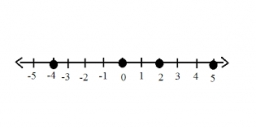# Increased 7062

I think the number. I will increase it by 6, then decrease the increased number by 12, and then multiply the result by the number -2. I divide the product by the number -8 and get the number -4. What number do I think?

x =  -10

### Step-by-step explanation:Did you find an error or inaccuracy? Feel free to write us. Thank you!

Tips for related online calculators
Do you have a linear equation or system of equations and looking for its solution? Or do you have a quadratic equation?